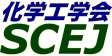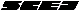## \$B^5-G09V1i(B

\$B:G=*99?7F|;~!'(B2012-08-20 15:02:17

### \$BM%=(O@J8>^(B

 \$BBh(B 1 \$BF|!&(BN \$B2q>l(B N113 \$B!VD69bB.2sE>%Y%k%+%C%WEIAu5!\$rBP>]\$H\$7\$?EICe8zN(\$rDc2<\$5\$;\$k0x;R\$N?tCM2r@OE*8!F\$!W(B (13:00\$B!A(B13:20) (\$BElKLBg1!9)(B) \$B0BB<(B \$B8wB@O:(B \$B;a(B \$B!&(B \$Bc7F#(B \$BBYMN(B \$B;a(B \$B!&(B \$B>1;R(B \$B@5OB(B \$B;a(B \$B!&(B \$B>>2<(B \$BMN2p(B \$B;a(B \$B!&(B \$B@DLZ(B \$B=(G7(B \$B;a(B \$B!&(B \$B;01:(B \$BN4Mx(B \$B;a(B \$B!&(B (\$BH,8M9)Bg(B) \$B>.3^86(B \$B?5(B \$B;a(B \$B!&(B \$BBg9u(B \$B@5IR(B \$B;a(B \$B!&(B (\$B90A0BgM}9)(B) \$B>kED(B \$BG@(B \$B;a(B \$B!&(B \$B0pB<(B \$BN4IW(B \$B;a(B \$BBh(B 1 \$BF|!&(BR \$B2q>l(B R113 \$B!V(BApplication of Ionic Liquids to Extraction Separation of Rare Earth Metals with an Effective Diglycol Amic Acid Extractant\$B!W(B (13:00\$B!A(B13:40) (\$B6eBg1!9)(B) \$B5WJ]ED(B \$BIY@8;R(B \$B;a(B \$B!&(B \$B2 \$B!&(B \$BGO>l(B \$BM:;0(B \$B;a(B \$B!&(B \$B>.Lx(B \$BM52p(B \$B;a(B \$B!&(B (\$B86;RNO5!9=(B) \$B2<>r(B \$B98;JO:(B \$B;a(B \$B!&(B (\$B6eBg1!9)(B) \$B?@C+(B \$BE5Jf(B \$B;a(B \$B!&(B \$B8eF#(B \$B2m9((B \$B;a(B \$BBh(B 1 \$BF|!&(BT \$B2q>l(B T115 \$B!V(BRelationship between Applied Static Magnetic Field Strength and Thermal Conductivity Values of Molten Materials Measured Using the EML Technique\$B!W(B (13:40\$B!A(B14:00) (\$BElKLBg1!9)(B) Baba Yuya \$B;a(B \$B!&(B Sugioka Ken-ichi \$B;a(B \$B!&(B Kubo Masaki \$B;a(B \$B!&(B Tsukada Takao \$B;a(B \$B!&(B (\$BElKLBgB?858&(B) Sugie Kazutoshi \$B;a(B \$B!&(B Kobatake Hidekazu \$B;a(B \$B!&(B Fukuyama Hiroyuki \$B;a(B \$BBh(B 2 \$BF|!&(BU \$B2q>l(B U209 \$B!VA/L@\$JN.L.@~\$N2D;k2=K!!W(B (11:40\$B!A(B12:00) (\$B:eBg1!4p9)(B) \$B66K\(B \$B=SJe(B \$B;a(B \$B!&(B \$B@>@n(B \$BC#:H(B \$B;a(B \$B!&(B \$B?7Ln(B \$B=c0l(B \$B;a(B \$B!&(B \$B@>B<(B \$BN<=S(B \$B;a(B \$B!&(B \$B0f>e(B \$B5AO/(B \$B;a(B

\$B9V1i%W%m%0%i%`(B
\$B2=3X9)3X2q(B \$BBh(B44\$B2s=)5(Bg2q(B(C) 2012 \$B8x1W
Most recent update: 2012-08-20 15:02:17
E-mail: inquiry-44fwww3.scej.org
This page was generated byeasp 2.28; proghtml 2.28c (C)1999-2011 kawase